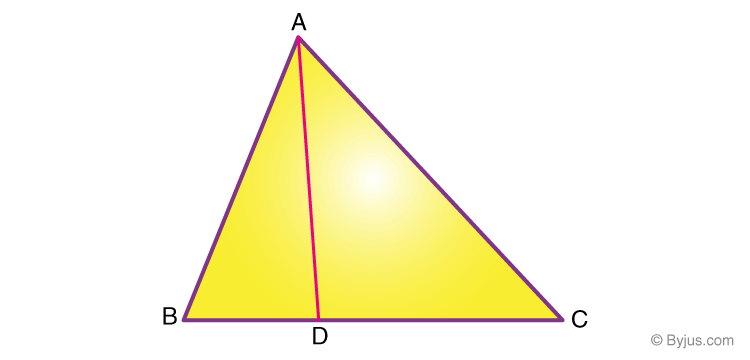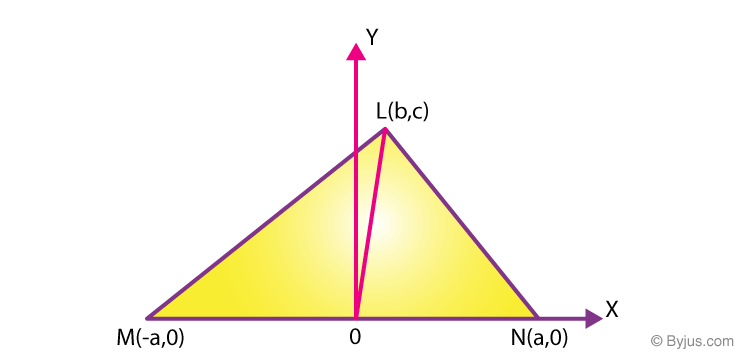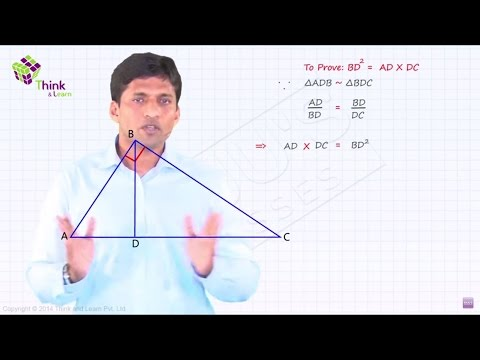Checkout JEE MAINS 2022 Question Paper Analysis : Checkout JEE MAINS 2022 Question Paper Analysis :

# Apolloniu's Theorem

In mathematics, theorems are the statements which have proven results based on the previously set statements, like theorems and generally confirmed statements like axioms. The theorems are known as the results which are proven to be accurate from the set of different axioms. This term is especially used in mathematics subject where these axioms are of numerical logic with the systems in the form of the question.## Statement and Proof of Apollonius’ Theorem

Medians are known to form the most important sets of the components in the geometry of triangles which are closely related to the triangle being independent of the geometric shapes. In Apollonius’ Theorem, the relation between the medians and the sides of the triangle are known. Apollonius’ theorem is a kind of theorem which relates to the length of the median of a triangle to the length of their sides.

## Apollonius’ Theorem Statement

Statement- “the sum of squares of any of the two sides of a triangle equals to twice its square on half of the third side, along with the twice of its square on the median bisecting the third side”

Or

If O is the midpoint of MN, one of the sides of the triangle (LMN), then prove that LN² + LM² = 2 {MO² + LO²}.## Apollonius’ Theorem Proof

Choose the origin of the rectangular form of the Cartesian coordinates at the point O and the x-axis coming along the sides MN and also OY as y – axis. If in case MN = 2a, then the coordinates of the points M, as well as N, are (a, 0) and (- a, 0) respectively. If coordinates of the point L are (b, c), then

LO² = (c – 0)² + (b – 0)² , (Since the coordinates of the point O are {0, 0})

=  c² + b²;

LM² =  (c – 0)² + (b + a) ² = c² + (a + b)²

MO² =  (0 – 0)² + (- a – 0)² = a²

also, LN² = (c – 0) ² + (b – a) ² = c² + (a – b)²

Therefore, LN²  + LM² = c² + (a + b) ² + c² + (b – a)²

= 2c² + 2 (a² + b²)

= 2(b² + c²) + 2a²

= 2LO² + 2MO²

= 2 (LO² + MO²).

= 2(MO² + LO²). {Hence Proved}